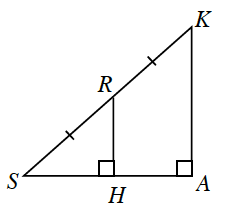### Home > GC > Chapter 7 > Lesson 7.3.1 > Problem7-103

7-103.Examine the diagram at right.

Draw $ΔSHR$ and $ΔSAK$ separately. Which angles are congruent?
2. What is the relationship between $HR$ and $AK$? Between $SH$ and $SA$? Between $SH$ and $HA$?
Compare $SR$ with $SK$. How are these lengths related? If the triangles are similar, then this relationship will be true for the other pairs of corresponding sides.
3. If $SK=20$ units and $RH=8$ units, what is $HA$?
Add the lengths to your diagram from part (a). If $SK=20$, what is $SR$?